Download Presentation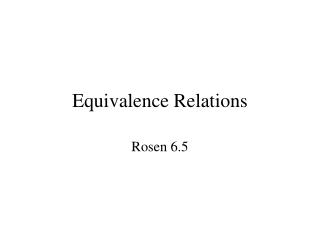Equivalence Relations

# Equivalence Relations - PowerPoint PPT Presentation

Equivalence Relations. Rosen 6.5. Some preliminaries. Let a be an integer and m be a positive integer. We denote by a MOD m the remainder when a is divided by m. If r = a MOD m, then a = qm + r and 0  r  m, qZ Examples Let a = 12 and m = 5, 12MOD5 = 2I am the owner, or an agent authorized to act on behalf of the owner, of the copyrighted work described.
Download Presentation## Equivalence Relations

An Image/Link below is provided (as is) to download presentation

Download Policy: Content on the Website is provided to you AS IS for your information and personal use and may not be sold / licensed / shared on other websites without getting consent from its author.While downloading, if for some reason you are not able to download a presentation, the publisher may have deleted the file from their server.

- - - - - - - - - - - - - - - - - - - - - - - - - - E N D - - - - - - - - - - - - - - - - - - - - - - - - - -
Presentation Transcript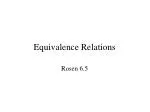### Equivalence Relations

Rosen 6.5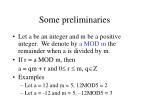Some preliminaries
• Let a be an integer and m be a positive integer. We denote by a MOD m the remainder when a is divided by m.
• If r = a MOD m, then

a = qm + r and 0 r  m, qZ

• Examples
• Let a = 12 and m = 5, 12MOD5 = 2
• Let a = -12 and m = 5, –12MOD5 = 3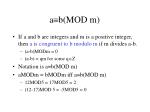ab(MOD m)
• If a and b are integers and m is a positive integer, then a is congruent to b modulo m if m divides a-b.
• (a-b)MODm = 0
• (a-b) = qm for some qZ
• Notation is ab(MOD m)
• aMODm = bMODm iff ab(MOD m)
• 12MOD5 = 17MOD5 = 2
• (12-17)MOD 5 = -5MOD5 = 0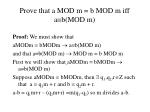Prove that a MOD m = b MOD m iff ab(MOD m)

Proof: We must show that

aMODm = bMODm  ab(MOD m)

and that ab(MOD m)  MOD m = b MOD m

First we will show thataMODm = bMODm  ab(MOD m)

Suppose aMODm = bMODm, then  q1,q2,rZ such that a = q1m + r and b = q2m + r.

a-b = q1m+r – (q2m+r) =m(q1-q2) so m divides a-b.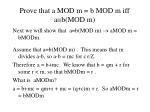Prove that a MOD m = b MOD m iff ab(MOD m)

Next we will show that ab(MOD m)  aMOD m = bMODm.

Assume that ab(MOD m) . This means that m divides a-b, so a-b = mc for cZ.

Therefore a = b+mc. We know that b = qm + r for some r < m, so that bMODm = r .

What is aMODm?

a = b+mc = qm+r + mc = (q+c)m + r. So aMODm = r = bMODm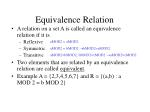Equivalence Relation
• A relation on a set A is called an equivalence relation if it is
• Reflexive
• Symmetric
• Transitive
• Two elements that are related by an equivalence relation are called equivalent.
• Example A = {2,3,4,5,6,7} and R = {(a,b) : a MOD 2 = b MOD 2}

aMOD2 = aMOD2

aMOD2 = bMOD2 bMOD2=aMOD2

aMOD2=bMOD2, bMOD2=cMOD2 aMOD2=cMOD2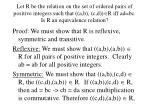Let R be the relation on the set of ordered pairs of positive integers such that ((a,b), (c,d))R iff ad=bc. Is R an equivalence relation?

Proof: We must show that R is reflexive, symmetric and transitive.

Reflexive: We must show that ((a,b),(a,b))  R for all pairs of positive integers. Clearly ab = ab for all positive integers.

Symmetric: We must show that ((a,b),(c,d)  R, the ((c,d),(a,b))  R. If ((a,b),(c,d)  R, then ad = bc -> cb = da since multiplication is commutative. Therefore ((c,d),(a,b))  R,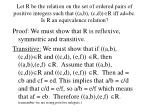Let R be the relation on the set of ordered pairs of positive integers such that ((a,b), (c,d))R iff ad=bc. Is R an equivalence relation?

Proof: We must show that R is reflexive, symmetric and transitive.

Transitive: We must show that if ((a,b), (c,d))Rand ((c,d), (e,f)) R, then ((a,b),(e,f) R. Assume that ((a,b), (c,d))Rand ((c,d), (e,f)) R. Then ad = cb and cf = ed. This implies that a/b = c/d and that c/d = e/f, so a/b = e/f which means that af = eb. Therefore ((a,b),(e,f) R. (remember we are using positive integers.)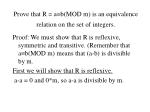Prove that R = ab(MOD m) is an equivalence relation on the set of integers.

Proof: We must show that R is reflexive, symmetric and transitive. (Remember that ab(MOD m) means that (a-b) is divisible by m.

First we will show that R is reflexive.

a-a = 0 and 0*m, so a-a is divisible by m.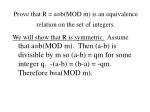Prove that R = ab(MOD m) is an equivalence relation on the set of integers.

We will show that R is symmetric. Assume that ab(MOD m). Then (a-b) is divisible by m so (a-b) = qm for some integer q. -(a-b) = (b-a) = -qm. Therefore ba(MOD m).Prove that R = ab(MOD m) is an equivalence relation on the set of integers.

We will show that R is transitive. Assume that ab(MOD m) and that bc(MOD m). Then  integers j,k such that (a-b) = jm, and (b-c) = km.

(a-b)+(b-c) = (a-c) = jm+km = (j+k)m

Since j+k is an integer, then m divides (a-c) so ac(MOD m).Equivalence Class

Let R be an equivalence relation on a set A. The set of all elements that are related to an element of A is called the equivalence class of a.

The equivalence class of a with respect to R is denoted [a]R. I.e., [a]R = {s | (a,s)  R}

Note that an equivalence class is a subset of A created by R.

If b  [a]R, b is called a representative of this equivalence class.Example

Let A be the set of all positive integers and let R = {(a,b) | a MOD 3 = b MOD 3}

How many equivalence classes (rank) does R create?

3Digraph Representation
• It is easy to recognize equivalence relations using
• digraphs.
• The subset of all elements related to a particular element forms a universal relation (contains all possible arcs) on that subset.
• The (sub)digraph representing the subset is called a complete (sub)digraph. All arcs are present.
• The number of such subsets is called the rank of the equivalence relationPartition
• Let S1, S2, …, Sn be a collection of subsets of A. Then the collection forms a partitionof A if the subsets are nonempty, disjoint and exhaust A.
• Si 
• SiSj =  if i  j
• Si = A

If R is an equivalence relation on a set S, then the equivalence classes of R form a partition of S.A has two elements

A has one element. One equivalence class, rank =1.

rank = 2

rank = 1A has three elements

Rank = 3

Rank = 2

Rank = 1A has four elements

Rank = 4

Rank = 1A has four elements

Rank = 2A has four elements

Rank = 2A has four elements

Rank = 3• 1 for n = 1
• 2 for n = 2
• 5 for n = 3
• 13 for n = 4
• ? for n = 5
• Is there recurrence relation or a closed form solution?